# GMAT Math : Calculating an angle of a line

## Example Questions

2 Next →

### Example Question #11 : Calculating An Angle Of A Line

What is the measure of an angle that is complementary to a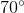angle?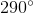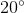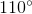Explanation:

Two angles are complementary if the total of their degree measures is. Therefore, an angle complementary to aangle measures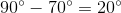### Example Question #12 : Calculating An Angle Of A Line

What is the measurement of an angle that is congruent to an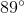angle?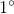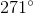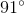Explanation:

Two angles are congruent if they have the same degree measure. Therefore, an angle congruent to anangle also measures### Example Question #11 : Calculating An Angle Of A Line

A right triangle is given with a missing value of. It is stated that the triangle is an acute right triangle with angles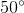and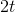. What is a possible value ofin degrees?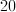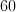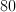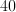Cannot be determinedExplanation:

It is important to recall that all triangles add to 180 degrees and a right triangle contains one angle that is equal to 90 degrees. Therefore, in this particular problem we can write the following equation to solve for the missing variable.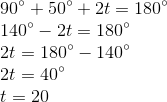2 Next →

### All GMAT Math Resources